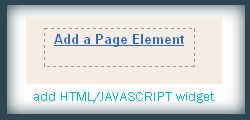Step 2 : In that Page Element window , choose HTML / JAVASCRIPT .

Step 3 : Now add this Javascript in that window.

‘;
}else{
html += ‘1‘;
}
}else{
html += ‘‘+ (p+1) +’ ‘;
}
}

if(eFlag ==0 && p == thisNum){
downPageHtml = ‘‘;
eFlag++;
}
}//end if(p>=(thisNum-displayPageNum-1) && p< (thisNum+displayPageNum)){
}//end for(var p =0;p< htmlMap.length;p++){

if(thisNum>1){
if(!isLablePage){
html = ‘‘+ firstPageWord +’ ‘+upPageHtml+’ ‘+html +’ ‘;
}else{
html = ”+labelHtml + firstPageWord +’ ‘+upPageHtml+’ ‘+html +’ ‘;
}
}

html = ‘

Page ‘+thisNum+’ of ‘+(postNum-1)+’: ‘+html;

if(thisNum< (postNum-1)){
html += downPageHtml;
html += ‘ ‘+endPageWord+’‘;
}

if(postNum==1) postNum++;
html += ‘

‘;

if(isPage isFirstPage isLablePage){
var pageArea = document.getElementsByName(“pageArea”);
var blogPager = document.getElementById(“blog-pager”);

if(postNum < = 2){
html =”;
}

for(var p =0;p< pageArea.length;p++){
pageArea[p].innerHTML = html;
}

if(pageArea&&pageArea.length>0){
html =”;
}

if(blogPager){
blogPager.innerHTML = html;
}
}

}

After Adding this Javascript you need to drag and drop the widget below the Blog Posts main widget. See this screen shot

In the code above you can edit the lines in red to your wish .

1 : var pageCount = 5;

The digit in red represents number of posts to be shown in single page. Change the digit to show as many pages you want.

for example :

In my blog I have put that value as 2 . In each page you can see only 2 posts .

2 : var displayPageNum = 3;

here the digit in red represents number of pages to be listed.

For example :

In my blog I have chosen 3 , then 3 pages will be shown.

That’s it now we have added Page Navigation menu widget to our blog successfully.